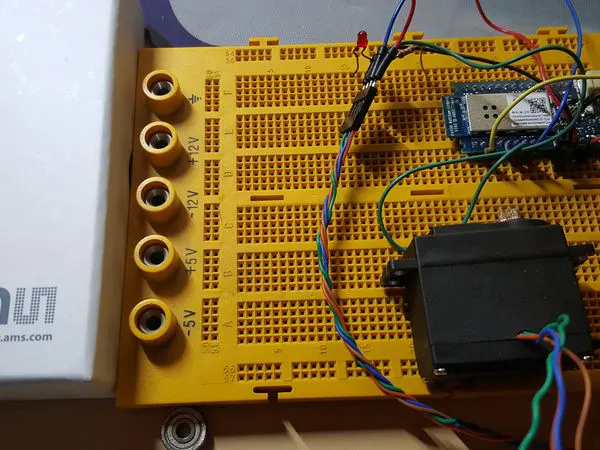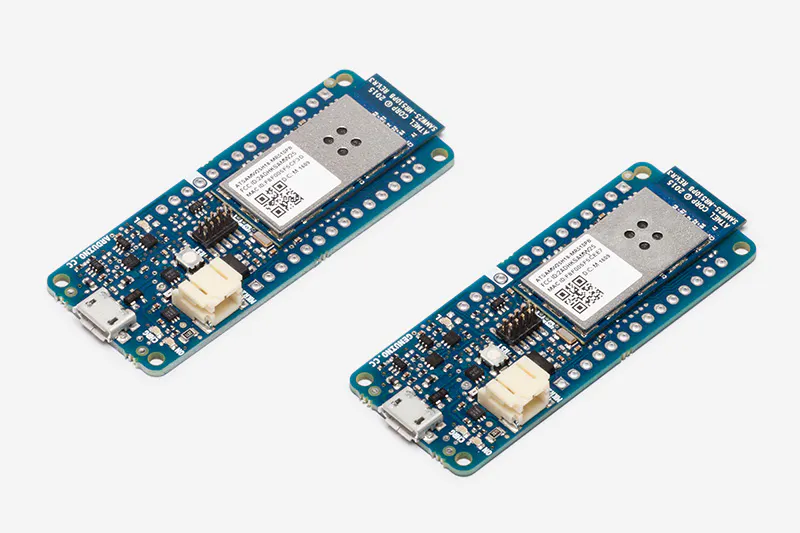Published © CC BY

# Control the Position of a Continuous Rotation Servo via WiFi

Position control using wifi from a smartphone sounds too good to be true? Do it with MKR1000.

IntermediateProtip8 hours7,305## Things used in this project

### Hardware componentsArduino MKR1000
×1

### Hand tools and fabrication machines3D Printer (generic)

## Schematics

### System diagram

Please note there is a magnet attached to the output shaft of the motor whose motion will be detected but the magnetic encoder chip.## Code

### dcservo_magencoder_UDP

C/C++
You can control a dolly or other carriage wirelessly.
```/* Miguel Sanchez 2106

This program uses an Arduino MKR1000 for a closed-loop control of a 360servo.
Motor motion is detected by a magnetic encoder.

MKR1000 Pins used:
I2C input is used for magnetic encoder SCL SDA
Digital output 7 is servo control
Please note PID gains kp, ki, kd need to be tuned to each different setup.
Values can later stored to flash with W command for persistent storage
*/

#include <FlashStorage.h>  // for PID configuration storage
#include <PID_v1.h>
#include <Wire.h>         // support for I2C encoder
#include <Servo.h>

#include <WiFi101.h>
#include <WiFiUdp.h>

const char* ssid = "****";          // your home SSID

// Create an instance of the server
// specify the port to listen on as an argument
WiFiUDP client;

Servo servo;

byte pos; int p = 0;
double kp = .3, ki = 0, kd = 0.01;
double input = 0, output = 0, setpoint = 0;
PID myPID(&input, &output, &setpoint, kp, ki, kd, REVERSE);
volatile long encoder0Pos = 0;
boolean auto1 = false, auto2 = false, counting = false;
long pos1;

long target1 = 0; // destination location at any moment

//for motor control ramps 1.4
bool newStep = false;
bool oldStep = false;
bool dir = false;
byte skip = 0;

{
word retVal = -1;

/* Read Low Byte */
Wire.beginTransmission(0x36);
Wire.write(0x0d);
Wire.endTransmission();
Wire.requestFrom(0x36, 1);
while (Wire.available() == 0);
int low = Wire.read();

/* Read High Byte */
Wire.beginTransmission(0x36);
Wire.write(0x0c);
Wire.endTransmission();
Wire.requestFrom(0x36, 1);

while (Wire.available() == 0);

word high = Wire.read();

high = high << 8;
retVal = high | low;

return retVal;
}

int angle, diff;
double before = 0;

void setup() {
Serial.begin (115200);
help();
recoverPIDfromEEPROM();
//Setup the pid
myPID.SetMode(AUTOMATIC);
myPID.SetSampleTime(1);
myPID.SetOutputLimits(0, 180);
Wire.begin(); // start I2C driver code D6 & D7
servo.attach(7);
servo.write(90);

// Connect to WiFi network
Serial.println();
Serial.println();
Serial.print("Connecting to ");
Serial.println(ssid);

while (WiFi.status() != WL_CONNECTED) {
delay(500);
Serial.print(".");
}
Serial.println("");
Serial.println("WiFi connected");

// Start the server
client.begin(23);
Serial.println("UDP started");

// Print the IP address
IPAddress ip = WiFi.localIP();
Serial.println(ip);

}
void loop() {
// process encoder rollover
diff = angle - before;
if (diff < -3500) pos1 += 4096;
else if (diff > 3500) pos1 -= 4096;
before = angle;
input = pos1 + angle;

//input = encoder0Pos;
setpoint = target1;
myPID.Compute();
if (Serial.available()) process_line();
if (client.parsePacket()) process_line_net();
pwmOut(output);
if (auto1) if (millis() % 3000 == 0) target1 = random(2000); // that was for self test with no input from main controller
if (auto2) if (millis() % 1000 == 0) printPos();
if (counting && abs(input - target1) < 15) counting = false;
if (counting &&  (skip++ % 5) == 0 ) {
pos[p] = input;
if (p < 999) p++;
else counting = false;
}

}

// continuous servo output
void pwmOut(int out) {
servo.write(out);
}

void process_line() {
char cmd = Serial.read();
if (cmd > 'Z') cmd -= 32;
switch (cmd) {
case 'P': kp = Serial.parseFloat(); myPID.SetTunings(kp, ki, kd); break;
case 'D': kd = Serial.parseFloat(); myPID.SetTunings(kp, ki, kd); break;
case 'I': ki = Serial.parseFloat(); myPID.SetTunings(kp, ki, kd); break;
case '?': printPos(); break;
case 'X': target1 = Serial.parseInt(); counting = true; for (int i = 0; i < p; i++) pos[i] = 0; p = 0; break;
case 'T': auto1 = !auto1; break;
case 'A': auto2 = !auto2; break;
case 'Q': Serial.print("P="); Serial.print(kp); Serial.print(" I="); Serial.print(ki); Serial.print(" D="); Serial.println(kd); break;
case 'H': help(); break;
case 'W': writetoEEPROM(); break;
case 'K': //eedump(); break;
case 'R': recoverPIDfromEEPROM() ; break;
case 'S': for (int i = 0; i < p; i++) Serial.println(pos[i]); break;
case 'M': pwmOut(Serial.parseInt()); break; // just ignore it unless you disable pwmOut in the main loop
}
while (Serial.read() != 10); // dump extra characters till LF is seen (you can use CRLF or just LF)
}

void process_line_net() {
char cmd = client.read();
if (cmd > 'Z') cmd -= 32;
switch (cmd) {
case 'P': kp = client.parseFloat(); myPID.SetTunings(kp, ki, kd); break;
case 'D': kd = client.parseFloat(); myPID.SetTunings(kp, ki, kd); break;
case 'I': ki = client.parseFloat(); myPID.SetTunings(kp, ki, kd); break;
case '?': client.beginPacket(client.remoteIP(), client.remotePort()); client.print(F("Position=")); client.print(input); client.print(F(" PID_output=")); client.print(output); client.print(F(" Target=")); client.println(setpoint); client.endPacket(); break;
case 'X': target1 = client.parseInt(); counting = true; for (int i = 0; i < p; i++) pos[i] = 0; p = 0; break;
case 'T': auto1 = !auto1; break;
case 'A': auto2 = !auto2; break;
case 'Q': client.beginPacket(client.remoteIP(), client.remotePort()); client.print("P="); client.print(kp); client.print(" I="); client.print(ki); client.print(" D="); client.println(kd); client.endPacket(); break;
case 'H': client.beginPacket(client.remoteIP(), client.remotePort()); help_net(); client.endPacket(); break;
case 'W': writetoEEPROM(); break;
case 'K': //eedump(); break;
case 'R': recoverPIDfromEEPROM() ; break;
case 'S': //for (int i = 0; i < p; i++) client.println(pos[i]); break;
case 'M': pwmOut(client.parseInt()); break; // just ignore it unless you disable pwmOut in the main loop
}
while (client.read() != 10); // dump extra characters till LF is seen (you can use CRLF or just LF)
}
void printPos() {
Serial.print(F("Position=")); Serial.print(input); Serial.print(F(" PID_output=")); Serial.print(output); Serial.print(F(" Target=")); Serial.println(setpoint);
}

void help() {
Serial.println(F("\nPID DC motor controller and stepper interface emulator"));
Serial.println(F("by misan"));
Serial.println(F("Available serial commands: (lines end with CRLF or LF)"));
Serial.println(F("P123.34 sets proportional term to 123.34"));
Serial.println(F("I123.34 sets integral term to 123.34"));
Serial.println(F("D123.34 sets derivative term to 123.34"));
Serial.println(F("? prints out current encoder, output and setpoint values"));
Serial.println(F("X123 sets the target destination for the motor to 123 encoder pulses"));
Serial.println(F("T will start a sequence of random destinations (between 0 and 2000) every 3 seconds. T again will disable that"));
Serial.println(F("Q will print out the current values of P, I and D parameters"));
Serial.println(F("W will store current values of P, I and D parameters into EEPROM"));
Serial.println(F("H will print this help message again"));
Serial.println(F("A will toggle on/off showing regulator status every second\n"));
}

void help_net() {
client.println(F("\nPID DC motor controller and stepper interface emulator"));
client.println(F("by misan - porting cured by Exilaus"));
client.println(F("Available serial commands: (lines end with CRLF or LF)"));
client.println(F("P123.34 sets proportional term to 123.34"));
client.println(F("I123.34 sets integral term to 123.34"));
client.println(F("D123.34 sets derivative term to 123.34"));
client.println(F("? prints out current encoder, output and setpoint values"));
client.println(F("X123 sets the target destination for the motor to 123 encoder pulses"));
client.println(F("T will start a sequence of random destinations (between 0 and 2000) every 3 seconds. T again will disable that"));
client.println(F("Q will print out the current values of P, I and D parameters"));
client.println(F("W will store current values of P, I and D parameters into EEPROM"));
client.println(F("H will print this help message again"));
client.println(F("A will toggle on/off showing regulator status every second\n"));
}

// these are really writing to Flash, but I keep the EEPROM name for the sake of being like the ones for other Arduino versions

// for Flash memory storage of PID settings ...
typedef struct {
double kp, ki, kd, cks;
} eeprom;

eeprom data;

FlashStorage(my_flash_store, eeprom);

void writetoEEPROM() { // keep PID set values in EEPROM so they are kept when arduino goes off
data.kp = kp;
data.ki = ki;
data.kd = kd;
data.cks = kp + ki + kd + 1.0; // add 1.0 to avoid initial all zeros value that would match always
my_flash_store.write(data);
Serial.println("\nPID values stored to FLASH");
}

void recoverPIDfromEEPROM() {
double cks = data.kp + data.ki + data.kd + 1.0;
double cksEE = data.cks;
if (cks == cksEE) {
Serial.println(F("*** Found PID values on FLASH"));
kp = data.kp;
ki = data.ki;
kd = data.kd;
myPID.SetTunings(kp, ki, kd);
}
else Serial.println(F("*** Bad checksum"));
}
```

### dcservo project

Some other variants controlling DC and brushless motors instead of servos.

## Credits

### Miguel Sánchez

1 project • 3 followers
Teaching Arduino to Arts students.